MORE IN Elements of Civil Engg. & Engg. Mechanics
VTU First Year Engineering (P Cycle) (Semester 1)
Elements of Civil Engg. & Engg. Mechanics
June 2013
Total marks: --
Total time: --
INSTRUCTIONS
(1) Assume appropriate data and state your reasons
(2) Marks are given to the right of every question
(3) Draw neat diagrams wherever necessary

1(a)(i) Geotechnical engineering involves the study of ?
A) Water
B) Soil
C) Air
D) All of these
1 M
A) Inside the city
C) Around the city
D) None of these
1 M
1(a)(iii) The part of civil engineering which deals with waste water and solid waste is called,
A) Water supply engineering
B) Geotechnical engineering
C)Sanitary engineering
D) Structural engineering
1 M
1(a)(iv) A bascule bridge is a,
A) Floating bridge
B) Arch bridge
C) Suspension bridge
D) Movable bridge
1 M
1(b) Write a note on role of civil engineer in infrastructural development.
10 M
1(c) Name the different types of roads as per Nagpur plan.
6 M

2(a)(i) Moment of a force can be defined as the product of force and ______. distance from the line of action of force to the moment center.
A) Least
B) Maximum
C) Any
D) None of these
1 M
2(a)(ii) Effect of force on a body depends on,
A) Direction
B) Magnitude
C) Position
D) All of these
1 M
2(a)(iii) The forces which meet at one point and have their line of action in different planes are called
A) Coplanar concurrent forces
B) Non coplanar concurrent forces
C) Non coplanar non concurrent forces
D) None of these
1 M
2(a)(iv) Couple means two forces acting parallel,
A) Equal in magnitude and in the same direction.
B) Not equal in magnitude but in the same direction .
C) Equal in magnitude but opposite in direction.
D) None of these
1 M
2(b) Define force and state its characteristics.
6 M
2(c) Determine the magnitude and direction of the resultant for the system of forces shown in Q2 (c). Use classical method.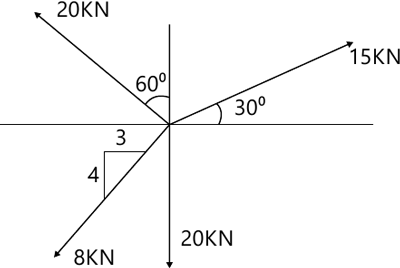10 M

3(a)(i) The technology of finding the resultant of a system of forces is called,
A) Resultant
B) Resolution
C) Composition
D) None of these
1 M
3(a)(ii) Equilibriant in nothing but a resultant,
A) Equal in magnitude and in the same direction.
B) Equal in magnitude but opposite in direction.-
C) Not equal in magnitude but in the same direction.
D) Not equal in magnitude and opposite in direction.
1 M
3(a)(iii) If two forces P and Q (P > Q),act on the same straight line but in opposite direction their resultant is
A) P + Q
B) P / Q
C) Q - P
D) P - Q
1 M
3(a)(iv) In coplanar concurrent force system if ?H = 0 then the resultant is
A) Horizontal
B) Vertical
C) Moment
D) None of these
1 M
3(b) State and prove Varignon's theorem of the moments.
6 M
3(c) Two spheres each of radius 100mm and weight 5kN is in a rectangular box as shown in fig. Calculate reactions at point of contacts.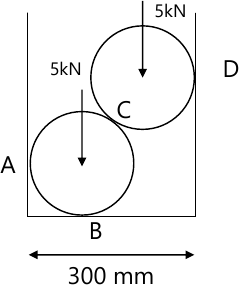10 M

4(a)(i) Moment of total area about its centroidal axis is
A) Twice the area
B) Three times the area
C) Zero
D) None of these
1 M
4(a)(ii) The centroid of a semicircle of radius R about its centroidal diametric axis is
A) 3R/4?
B) 3R/8?
C) 4R/?
D) 4R/3?
1 M
4(a)(iii) An axis over which one half of the plane figure is just mirror of the other half which is
A) Bottom most axis of the figure
B) Axis of symmetry
C) Unsymmetrical axis
D) None of these
1 M
4(a)(iv) Centroid of a rectangle of base width b and depth d is
A) b/3 end d/3
B) b/2 and d/2
C) b/4 and d/4
D) None of these.
1 M
4(b) Determine the centroid of a triangle by the method of integration.
6 M
4(c) Locate the centrid of the lamina shown in fig with respect to point O.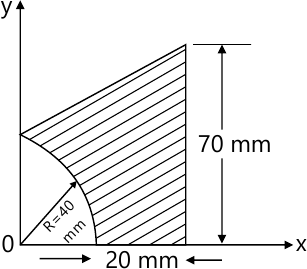10 M

5(a)(i) The necessary condition of equilibrium of a coplanar concurrent three system is algebric sum of ??? must be zero.
A) Horizontal and vertical threes
B) Moment of threes
C) Horizontal. vertical and moment of forces
D) None of these
1 M
5(a)(ii) In non concurrent force system if ??H=0, ??V=0 then the resultant is
A) Horizontal
B) Vertical
C) Moment
D) Zero
1 M
5(a)(iii) The force which is equal and opposite to the resultant is
A) Resultant force
B) Force
C) Equilibriant
D) None of these
1 M
5(a)(iv) The procedure of resolution is
A) To find the resultant of the force system
B) To break up an inclined force into two components
C) To find the equilibriant
D) None of these
1 M
5(b) Determine the reaction at the point ofshown in contact for the sphere shown in fig.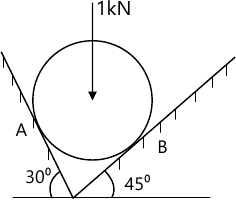6 M
5(c) Determine the angle ? for the system of strings ABCD in equilibrium as shown in fig.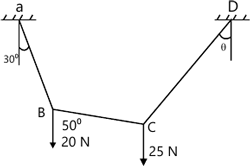10 M

6(a)(i) Statically determinate beams are,
A) The beams which can be analyzed completely using static equations of equilibrium
B) The beams which can be without using static equations of equilibrium
C) Fixed beams
D) None of these
1 M
6(a)(ii) Fixed beams are,
A) One end is fixed and the other is simply supported
B) Both ends are fixed
C) Both ends are roller supported
D) One end is fixed and the other is free.
1 M
6(a)(iii) The number of reaction components at fixed end of a beam are,
A) 2
B) 3
C) 4
D) None of these
1 M
6(a)(iv) U.D.L. stands for
D) All of these
1 M
6(b) Explain different types of supports.
6 M
6(c) Determine the reaction at the support for the beam shown in fig.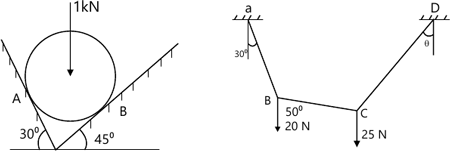10 M

7(a)(i) Angle of friction is angle between
A) the incline and horizontal
B) the normal reaction and friction force
C) the weight of the body and the friction force
D)Normal reaction and the resultant.
1 M
7(a)(ii) The force of friction developed at the contact surface is always
A) Parallel to the plane and along the direction of the applied force
B) Perpendicular to the plane
C) Parallel to the plane and opposite to the direction of the motion
D) All of these.
1 M
7(a)(iii) The maximum inclination of the plane on which the body free from external forces can repose is called
A) Cone of friction
B) Angle of friction
C) Angle of repose
D) None of these
1 M
7(a)(iv) The force of friction depends on
A) Area of contact
B) Roughness of the surface
C) Both area of contact and roughness of the surface
D) None of these.
1 M
7(b) State the laws of static friction.
4 M
7(c) A uniform ladder of length 15m and weight 750N rests against a vertical wall making an angle of 60° with the horizontal. Co-efficient of friction between the wall and the ladder is 0.3 and between the ground and the ladder is 0.25. A man weighing 500N ascends the ladder. How long will he be able to go before the ladder slips?
12 M

8(a)(i) The unit of radius of Gyration is
A) mm
B) mm2
C) mm3
D) mm4
1 M
8(a)(ii) The moment of inertia of an area about an axis which is in a plane perpendicular to the area is called
B) Polar moment of inertia
C) Second moment of area
D) None of these
1 M
8(a)(iii) The moment.of inertia ofa circle with 'd' as its diameter about its centroidal axis is
A) (?D2)/32
B) (?D2)/64
C)(?D4)/32
D) (?D4)/64
1 M
8(a)(iv) The moment of inertia of a square of side b about an axis through its centroid is
A) b4/12
B)b4/8
C) b4/36
D) b3/12
1 M
8(b) State and prove parallel axis theorem.
6 M
8(c) Find the moment of inertia of the region shown in Fig. about horizontal axis AB and also find the radius of Gyration about the same axis.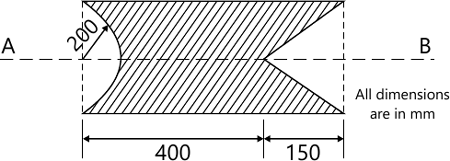10 M

More question papers from Elements of Civil Engg. & Engg. Mechanics